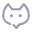# PHP 本周一开始的时间戳，周日结束的时间戳算法

fashion&super
2021-04-09 12:202304

``````\$today = strtotime('today'); // 今天
\$day = date('w', \$today);
\$begin = strtotime('-'. (\$day+6)%7 .'days', \$today);
\$end = strtotime('+'. (7-\$day)%7 .'days', \$today)+86399;``````

``````// \$time = strtotime('2021-04-3'); 指定某天
\$time = strtotime('today'); // 今天

\$week = date('w', \$time);
if (\$week == 0) {
\$week_start = mktime(0, 0, 0, date('m', \$time), date('d', \$time) - 6, date('Y', \$time));
\$week_end = mktime(23, 59, 59, date('m', \$time), date('d', \$time), date('Y', \$time));
} else {
\$week_start = mktime(0, 0, 0, date('m', \$time), date('d', \$time) - (\$week - 1), date('Y', \$time));
\$week_end = mktime(23, 59, 59, date('m', \$time), date('d', \$time) + (7 - \$week), date('Y', \$time));
}``````

``````// \$time = strtotime('2021-04-3'); 指定某天
\$time = strtotime('today'); // 今天

\$week = date('w', \$time);
\$week_start = mktime(0, 0, 0, date('m', \$time), date('d', \$time) - ((7 + ((\$week % 7) - 1)) % 7), date('Y', \$time));
\$week_end = mktime(23, 59, 59, date('m', \$time), date('d', \$time) + ((7 - \$week) % 7), date('Y', \$time));``````

0 条讨论